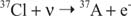## Solar Neutrino Problem

Our understanding of the central region of the Sun is based solely on theoretical calculations. In searching for a means of testing whether these calculations were correct, scientists realized that the neutrinos coming from the nuclear reactions would allow such a test. Neutrinos are very elusive particles. Originally believed to be massless, they travel essentially at the speed of light and have an extremely low likelihood of ever reacting again with other forms of matter. The boron to beryllium reaction in the proton‐proton cycle, however, has a special significance for scientists on Earth; this neutrino is a high‐energy neutrino that has the greatest possibility of being experimentally detected at Earth via the reactionMeasurement of the number of these neutrinos arriving at Earth then allows deduction of the number of reactions occurring in the core of the Sun. Because the reaction rate is very temperature dependent, astronomers have, in essence, a means of determining the true central temperature of the Sun to compare with the prediction of the Standard Solar Model. In an experiment begun in the 1960s, 100,000 gallons of the cleaning fluid perchloroethylene (C 2Cl 4; containing 2 × 10 30 atoms of 37Cl) has been used as the neutrino detector: a few chlorine atoms in the molecules of the cleaning fluid interact with solar neutrinos to produce argon. One then recovers the argon atoms from the tank to determine how many argon atoms are produced in each time period. The expected capture rate of neutrinos for the Standard Solar Model is 1 neutrino per day! After 30 years of operation, the actual capture rate is only one‐third to one‐half of this, a result that if correct could actually rule out the proton‐proton cycle as the source of the Sun's energy. Newer measurements of solar neutrinos, in a variety of other experiments that also detect other neutrinos from the thermonuclear reactions, have confirmed the apparent deficiency of the solar neutrino flux. But three decades of theoretical effort have found no satisfactory modification of the Standard Solar Model that can account for a lower number of neutrinos.

At present, it appears that the problem has not to do with understanding of the Sun but rather with the physics of neutrinos. Neutrinos exist in three forms (each associated with a specific of nuclear particle): electrons, muons, and tauons. If neutrinos actually have a tiny amount of mass (the original theory predicted that they were massless), then solar neutrinos can change. They leave the Sun as electron neutrinos, but in the eight minutes of travel between the Sun and Earth, they become equal numbers of the three neutrino types. Additional experiments are underway to test the hypothesis that neutrinos do have mass.